## Characterization of a submarine landslide in Santa María del Oro lake using a numerical model

Diego A. Pantoja, Anatoliy Filonov, Noel Gutiérrez

Departamento de Física

Universidad de Guadalajara, CUCEI

Direccion postal

Guadalajara, Mex. 44430

e-mail: diego.pantoja@academicos.udg.mx,

afilonov@prodigy.net.mx,

nogutier@ucsd.edu

## Abstract

During summer of 2016 an event was register in the volcanic lake of Santa María del Oro, a perturbation that triggers a considerable rise of amplitude on the lake system for at least two days. Due to the scarce observations in the lake, yet it is not definitive what event took place, a storm, a landslide or other. Based on numerical modelling a series of experiments were carry out in order to determine the kind of forcing that created those anomalies. This study is focused in characterizing a submarine landslide and the effects of the wind valley breeze. The results indicate that the lake acts as a dynamical membrane resonating to frequencies at 3.2 min (external seiche) and in a band of the 2-8 hrs (internal seiches) with the 2.64 hrs the most intense. After every forcing the water starts to oscillate uniformly and in a clockwise sense until the energy is dissipated by friction at the internal seiches frequencies.

KeyWords. Internal and external Seiches, Crater Lake, Delft3D model.

## INTRODUCTION

Long standing waves oscillations in enclosed water bodies, or seiches , are forced movements provoked mainly by atmospheric (storms) or topographic (seismic motion) perturbations. These oscillations that can span from a few minutes to several hours strongly depend on the shape of the basin and less in the intensity of the disturbed processes. The modal structure of the membrane-like movements losses its energy by dissipating mechanisms until the system came at rest if the external forcing ceases, . In volcanic lakes those oscillations are typically caused by wind burst or other situation like landslides due to the conic-shape of the basin. In august 18 of 2006 a device deployment in the Lake Santa Maria del Oro recorded a sudden perturbation on the background ambient configuration for a pair of days. In order to shed some light in what could be happened, the numerical approach was used to simulated the typical behaviour of the lake due to an external rapid forcing. The lake of Santa Maria del Oro, (21$^{\circ }$ 22' N and 104$^{\circ }$ 34' W, Nayarit, México), is a volcanic lake located 750 m over sea level, with nearly a circular shape of $\sim$ 2 km of diameter and a maximum depth of 65 m near the center. The lateral slopes are pronounce, except at the west coast where they end smoothly at a bay, Fig. 1. The atmospheric forcing of the lake comprises a valley breeze circulation due to the mountain chain of 200-300 m high that runs east-west in the northern and southern section of the region. At daylight the wind blows to the west and at night time the wind blows to east , Fig.1. The summer stratification of the lake range from 29 $^{\circ }$C on the surface to 22 $^{\circ }$C at the bottom. The thermocline (where there is an abrupt jump of temperature) lies around 15 m deep , Fig. 1.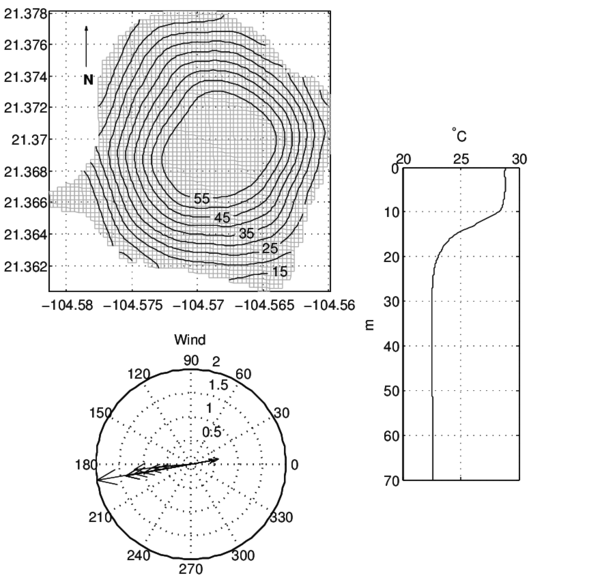Figure 1: Bathymetry of Santa María del Oro Lake [m] (upper), temperature profile for summer (right) and typical wind valley breeze (bottom).

According to , a possible landslide took place near the bay at the western section of the lake during august of 2006. The observed perturbations co-oscillated with the background system with a periods between 2 and 6 hrs.

## MODEL

Delft3D is an open-source numerical model developed by WL/Delft Hydraulics and the Delft University of Technology . It includes implementations of several mathematical models for different physical phenomena (currents, transport, wave propagation, morphological developments, etc.). In the present study, it is used to predict the circulation in a lake system. The Delft3D model solves the Navier-Stokes equations for an incompressible fluid, under the shallow water and the Boussinesq assumptions using a finite difference scheme (see ). The model includes the depth-averaged horizontal momentum equations, (shown here in Cartesian coordinates for the sake of clarity):

 ${\frac {\partial u}{\partial t}}+\mathbf {u} \cdot \nabla u+g{\frac {\partial \eta }{\partial x}}-fv+{\frac {u\Vert \mathbf {u} \Vert }{C^{2}(d+\eta )}}-{\frac {F_{x}}{\rho (d+\eta )}}-\nu \nabla ^{2}u=0,$ (1) ${\frac {\partial v}{\partial t}}+\mathbf {u} \cdot \nabla v+g{\frac {\partial \eta }{\partial y}}-fu+{\frac {v\Vert \mathbf {u} \Vert }{C^{2}(d+\eta )}}-{\frac {F_{y}}{\rho (d+\eta )}}-\nu \nabla ^{2}v=0,$ (2)

the depth-averaged continuity equation:

 ${\frac {\partial \eta }{\partial t}}+{\frac {\partial (d+\eta )u}{\partial x}}+{\frac {\partial (d+\eta )v}{\partial y}}=Q(d+\eta ),$
(3)

and the vertical momentum equation, which reduces to the hydrostatic pressure relationship via the Boussinesq approximation:

 ${\frac {\partial p}{\partial z}}=-\rho g.$
(4)

where $u,v$ are the depth-averaged velocity in the $x$ and $y$ directions, $C$ is the Chézy coefficient, $d$ is the water depth, $\eta$ is the free surface elevation above the reference plane (at $z=0$), $\mathbf {u}$ is a two dimensional current vector, whose Euclidean norm is $\Vert \cdot \Vert$ , $Q$ are sinks or sources of water, $f$ is Coriolis force, $F_{x,y}$ is Reynolds stress, $g$ is the gravity, $\nu$ is the horizontal eddy viscosity, $p$ is the pressure and $\rho$ the water density. In the horizontal direction, the model uses orthogonal curvilinear grids, which support both cartesian and spherical coordinates. Both coordinate systems permit the management of geometrically complex domains. In the vertical direction, Delft3D uses the $\sigma$ or the z coordinate systems. The z coordinate system is made up of layers with predefined depths. In this particular study, the spherical and z-coordinate system are used without boundary conditions. All the simulations were ran for only six days within a mesh of 156 x 126 number of cell in the (x,y)-directions, respectively, $dx\sim dy\sim 16$ m, with 20 z-layer in the vertical distributed according to [10 6 4 3 2] % of the water column, where the first value is assigned to the bottom layer and the last value is assigned to the upper layer (surface). In every cell the number of layers is variable depending on the water depth. The time step used was $\Delta t=3$ seg.

## MODEL RESULTS

In order to investigate the possible causes that appears in those of the observational data, three scenarios were set-up for the early stage of the perturbation. A Landslide-forced simulation (Ls), a Wind-forced simulation (Ws), and a Landslide- and Wind-forced simulation (LWs). The Ls is a configuration that characterizes a landslide which comprise an equivalent flux of water displaced by the sediment. Remembering that the intensity of the forcing is not as important as the geometrical shape of the basin, then the input of energy here was chosen rather arbitrary. The Ws is the typical configuration as the lake develops day by day in response of the valley breeze wind, and the LWs is the combination of both forcing. The numerical event (landslide) for the Ls was configured to happen at hr 1 in the day 2. After the impulsive forcing the water level was set in motion in a back- and forward movement rotating counter-clockwise, Fig. 2.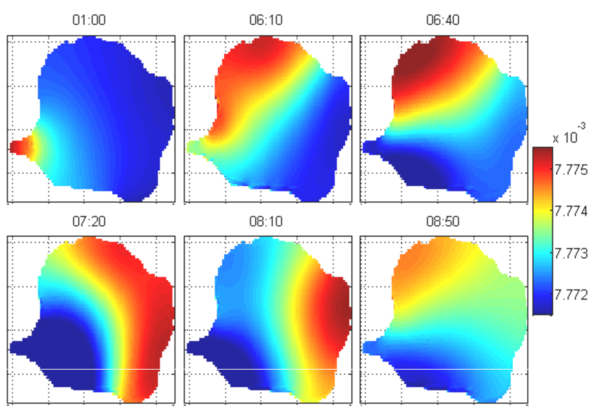Figure 2: Snapshots of water level six hours later of the simulated landslide. Without considering the first frame, the period expand nearly three hours of simulation. Color represent water level in m.
The system was moving almost by a day-long and then goes back to the state of rest. During the first three hrs the water level was moved with a higher frequency, (Fig 3, upper panels), and then after the third hr, the system damps by 2 orders of magnitude reducing also its frequency considerably, (Fig 3, lower panels). In the east-west section the motion seems more regular and more intense than in the north-south section. However, in both sections the water level shows an opposite behaviour, while in one side the water level is upward and in the other side is downward, resembling the movement show in Fig.2.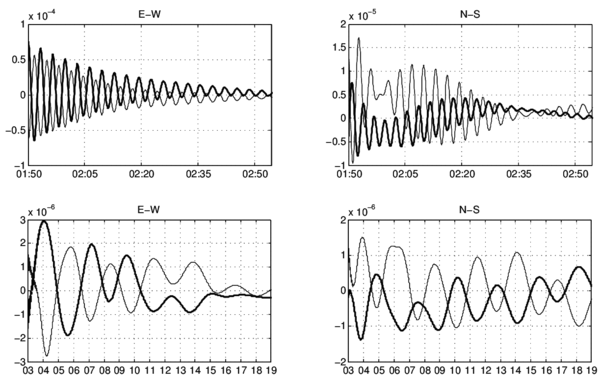Figure 3: Time series of water level at four observation points. In the upper panel is shown the early development of the water level at the east-west points (left) and at the north-south (right) points, note the x-axis is in minutes. At the lower panel are the corresponding sections through the time evolution during the next 19-hours after three hours the initial perturbation. In this case, the time series is in hours. The thick-line represent the eastern and southern points. Note the change in scales in every plot.
With respect to the currents, they behave similar as the water level. The currents are nearly homogeneous in direction but varying cyclonically and lowering its magnitude as the time progress, Fig 4. It also can be seen that in surface layers the circulation is more regular than in lower layers.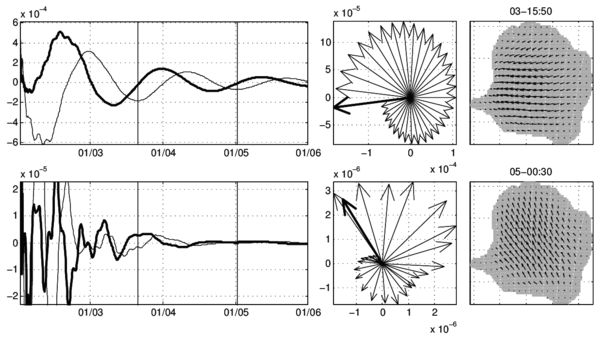Figure 4: Horizontal velocity components at the surface layer (top) and at the sixth layer in the bottom of the lake (bottom). In every row, is shown the time series of the components (left), a representative diagram of the vector velocity (center) and the initial snapshot of the horizontal velocity field (right). The thick line in time series represent the north-south component, the vertical lines the initial time marked as in the first panel whereas the thick vector represents the initial time.
In order to characterized the whole variability of the lake, the power spectra was computed for the water level time series. This technique was used successfully in others lakes (see e.g. [6,7]). In Fig. 5, are shown the spectrum of several points in the lake. As can be seen, the motion is concentrated into the frequency of the 3.2 min, and in the frequency band of 2-8 hrs around 2.64 hrs.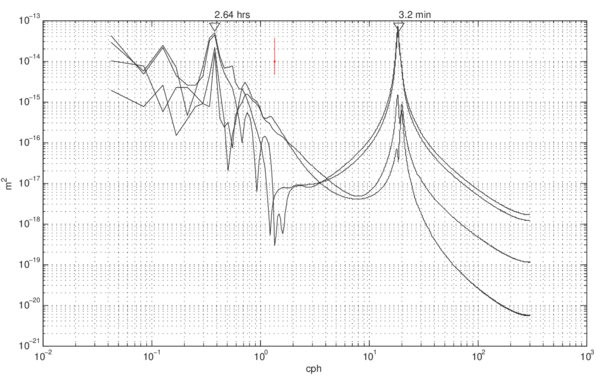Figure 5: Power spectra of the water level at the four points in the lake. The upper lines represent the east-west points, whereas the lower lines the north-south points. The vertical line correspond to the 95% confidence interval. The triangles mark the 2.64 hrs and 3.2 min frequencies.
In Fig. 6, are shown the power spectra of the simulation Ws and LWs. The interesting fact is that when the lake is forced only with wind (Ws) again the motion is set in the band of the 2-8 hrs (black lines), whereas in the combined simulation appears that both frequencies can coexist (blue lines).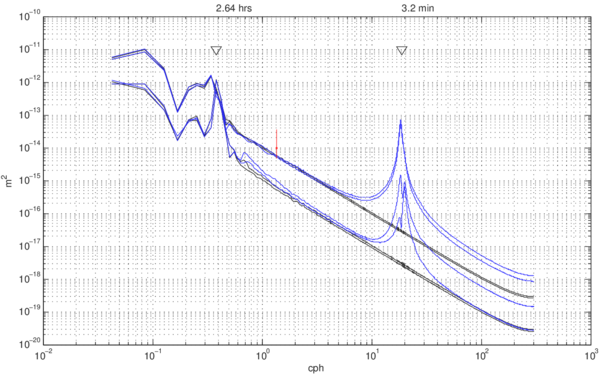Figure 6: Same as Fig. 5, for Ws (Black lines) and LWs (Blue lines).

## SUMMARY AND DISCUSSION

Lake Santa María del Oro can be considered as a circular basin which according to the 2D structure modal configuration, obeys the following standing oscillation from the seiches motion ():

 $\zeta (x,y,t)=J_{s}(kr)[A_{s}cos(s\theta )+B_{s}Sin(s\theta )]cos\omega t,$
(5)

For a basin with radius r, where $x=rcos\theta$, $y=rsin\theta$ and $\theta$ the polar angle. $J_{s}(kr)$ is the Bessel function of order s, $A_{s}$ and $B_{s}$ are arbitrary constants and $s=0,1,2,3,...$, with the roots of $J_{s}(ka)$ given the normal modes of the system. The period of oscillation of the first external model correspond to $T=2\pi /k{\sqrt {gH}}$, where g is the gravity and H the mean depth. If the lake was considered homogeneous in temperature, the first mode of oscillation (external mode) will remain between 2-3 min, whereas for an stratified lake, the first internal mode remains between 2.1-4.5 hrs ([2,8]). As shown by the combined configuration (Fig. 6), the resonant internal modes can coexist in the same simulation, and in the same band of frequency (2-8 hrs), so there is not a very clear contribution of which forcing caused the sudden perturbation on the observational data. Both simulations, wind and submarine landslide, can cause resonant amplification on the lake. Then, with the information at hand from the numerical outputs we can not confirm that effectively was a landslide that perturb the lake system, there is more work to do in order to support the idea of a topographical perturbation.

## Acknowledgements

This work was supported by the Mexican Secretaría de Educación Publica (SEP) through the Program for Teachers PRODEP of DAP. The AF acknowledge the support of CONACyT.

Back to Top

### Document informationPublished on 17/11/17
Submitted on 16/11/17

Volume 1, 2017
Licence: CC BY-NC-SA license

### Document Score0

Views 30
Recommendations 0

### Keywords